IMO Shortlist 2014 problem G6

Kvaliteta:
Avg: 0,0
Težina:
Avg: 8,0

Let$ABC$ be a fixed acute-angled triangle. Consdeir some points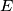$E$ and$F$ lying on the sides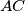$AC$ and$AB$, respecitvely, and let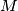$M$ be the midpoint of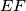$EF$. Let the perpendicular bisector of$EF$ intersect the line$BC$ at$K$, and let the perpendicular bisector of$MK$ intersect the lines$AC$ and$AB$ ant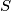$S$ and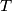$T$, respectively. We call the pair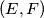$(E, F)$ interesting, the quadrilateral$KSAT$ is cyclic.

Suppose that the pairs$(E_1, F_1)$ and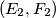$(E_2, F_2)$ are interesting. Prove that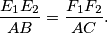(Iran)

Izvor: https://www.imo-official.org/problems/IMO2014SL.pdf